# 1st PUC Chemistry Series-3

#### PUC Science 1st Year Chemistry Series-3 quiz

0
Created on Bywefru

1st PUC Chemistry Series 3

1 / 10

Q21 Which of the following aqueous solution will be the best conductor of electricity?

2 / 10

Q22 . In 0.10 M aqueous solution of pyridine (C5H5N), find the percentage of pyridine that forms pyridinium ion (C5H5N+H) (Kb for C5H5N = 1.7 x 10-9 )

3 / 10

Q.23

Find the equilibrium constant of the reaction

If the equilibrium constant for the following reactions are given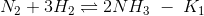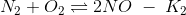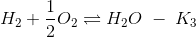4 / 10

Q.24 5. Highest pH will be recorded for which of the following solutions if they are equimolar

5 / 10

Q.25

The equilibrium constant is 278 for the reaction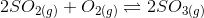at the same temperature, what will be the equilibrium constant for the following reaction?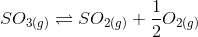6 / 10

Q.26 7. What will be the pH of a buffer solution having an equal concentration of B and HB (Kb = 10-10 for B)

7 / 10

Q.26 7. What will be the pH of a buffer solution having an equal concentration of B and HB (Kb = 10-10 for B)

8 / 10

Q.28On increasing the concentration of reactants in a reversible reaction, then equilibrium constant will

9 / 10

Q.29Find the conjugate acid of NH2

10 / 10

Q.30 Find the successive elements of the periodic table with ionisation energies, 2372, 520 and 890 kJ per mol respectively